# X-ray transform

In 1963, A.M. Cormack introduced a powerful diagnostic tool in radiology, computerized tomography, which is based on the mathematical properties of the X-ray transform in the Euclidean plane [a1] (cf. also Tomography). For a compactly supported continuous function, its X-ray transformis a function defined on the family of all straight linesin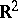as follows: let the unit vectorrepresent the direction ofand letbe its signed distance to the origin, so thatis represented by the pair(as well as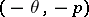); then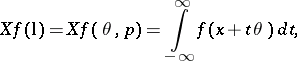whereis an arbitrary point on the line. This transform had already been considered in 1917 by J. Radon, who found its inverse with the help of its adjoint, given by the average valueof theover the family of all lineswhich are at a (signed) distancefrom the point, namely,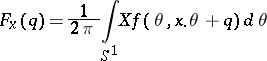where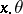is the Euclidean inner product betweenand. Radon then showed that the functioncan be recovered by the formula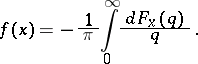The generalization of the X-ray transform to Euclidean spaces of arbitrary dimension and replacing the family of all lines by the family of all affine subspaces of a fixed dimension is known as the Radon transform [a1]. For the Radon transform in the broader context of symmetric spaces, see also [a2].

Note that the adjoint of the X-ray transform can be traced back to the Buffon needle problem (1777): find the average number of times that a needle of length, dropped at random on a plane, intersects one of the lines of a family of parallel lines located at a distance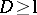(cf. also Buffon problem). As explained in [a3], Chapt. 5, the solution leads to the consideration of a measureon the space of all lines in the plane and ofinvariance under all rigid motions. This measure induces a functionalon the family of compact setsby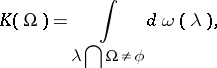which is basically the adjoint of the X-ray transform. Thus, among the generalizations of the X-ray transform and its adjoint, one also finds basic links to integral geometry [a3], [a6], combinatorial geometry [a4], convex geometry [a5], as well as the Pompeiu problem.